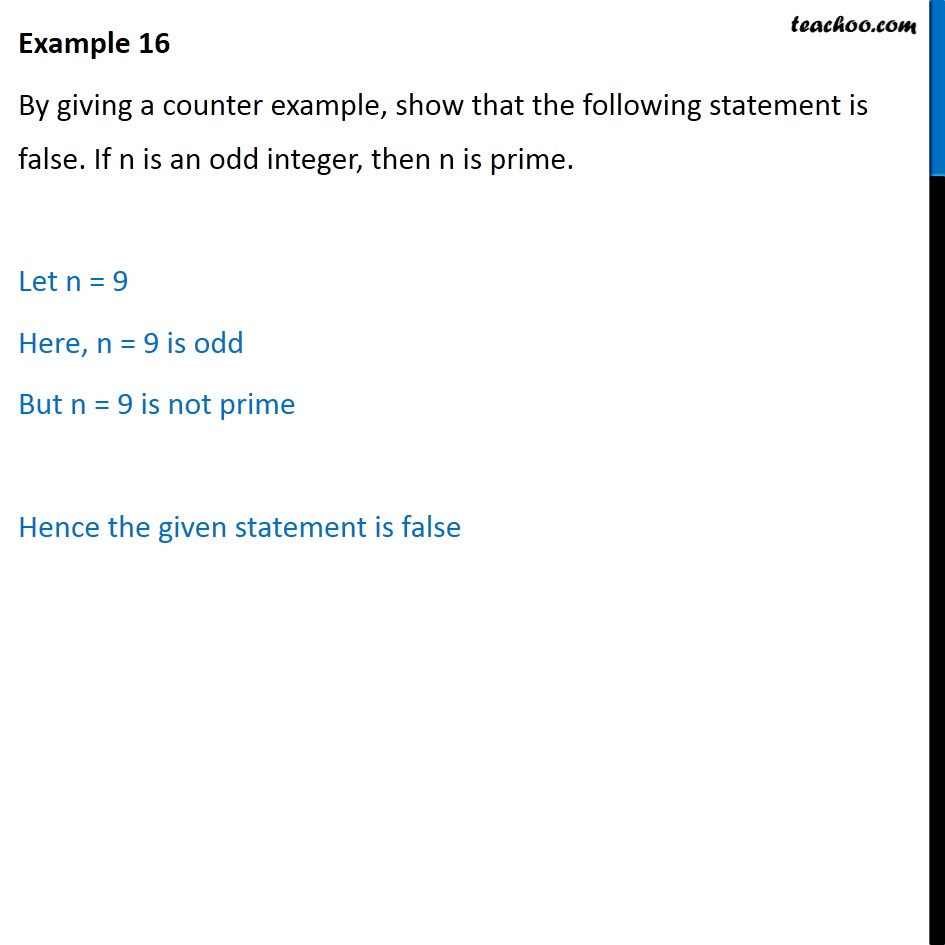1. Chapter 14 Class 11 Mathematical Reasoning (Deleted)
2. Concept wise
3. Proving not true/false (by giving counter examples)

Transcript

Example 16 By giving a counter example, show that the following statement is false. If n is an odd integer, then n is prime. Let n = 9 Here, n = 9 is odd But n = 9 is not prime Hence the given statement is false